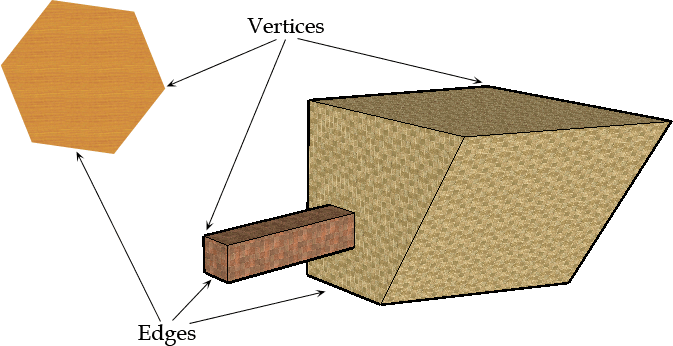SEARCH HOMEMath Central Quandaries & QueriesQuestion from Sophie, a student: I don't understand what is a vertex and what is an edge.Please put a picture up and explain. I am a fifth grader.Hi Sophie.

Here is a drawing showing you a flat hexagon shape and an odd 3-D shape.Vertices is the plural form of the word vertex.

A vertex is a single point. It joins edges together in a shape. Often, we also might call it a corner or just a point.

An edge is a line segment. It connects vertices together. In a two-dimensional object like the hexagon above, we sometimes call it a side.

A flat surface (like the hexagon itself, or any of the flat sides of the 3-D figure on the right) is usually called a face, but sometimes we call it a side too. That's why we usually try to avoid the word side when working with 3-D objects because it is less clear than using the terms edge and face instead.

There is a fascinating mathematical relationship between the number of vertices, edges and faces that was discovered by a famous Swiss mathematician named Leonhard Euler (it is pronounced like "oiler"). If you are interested in learning more, we have many other articles about this that you can find by typing Euler into our Quick Search.

Hope this helps,
Brennan Yaremko and Stephen La Rocque.Math Central is supported by the University of Regina and The Pacific Institute for the Mathematical Sciences.